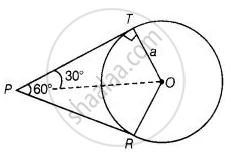# If angle between two tangents drawn from a point P to a circle of radius a and centre O is 60°, then OP = a3 - Mathematics

MCQ
True or False

If angle between two tangents drawn from a point P to a circle of radius a and centre O is 60°, then OP = asqrt(3)

• True

• False

#### Solution

This statement is True.

Explanation:From point P, two tangents are drawn

Given, OT = a

Also, line OP bisects the ∠RPT.

∴ ∠TPO = ∠RPO = 30°

Also, OT ⊥ PT

In right-angled ΔOTP,

sin 30^circ = (OT)/(OP)

⇒ 1/2 = a/(OP)

⇒ OP = 2a

Concept: Concept of Circle - Centre, Radius, Diameter, Arc, Sector, Chord, Segment, Semicircle, Circumference, Interior and Exterior, Concentric Circles
Is there an error in this question or solution?

#### APPEARS IN

NCERT Mathematics Exemplar Class 10
Chapter 9 Circles
Exercise 9.2 | Q 6 | Page 105
Share